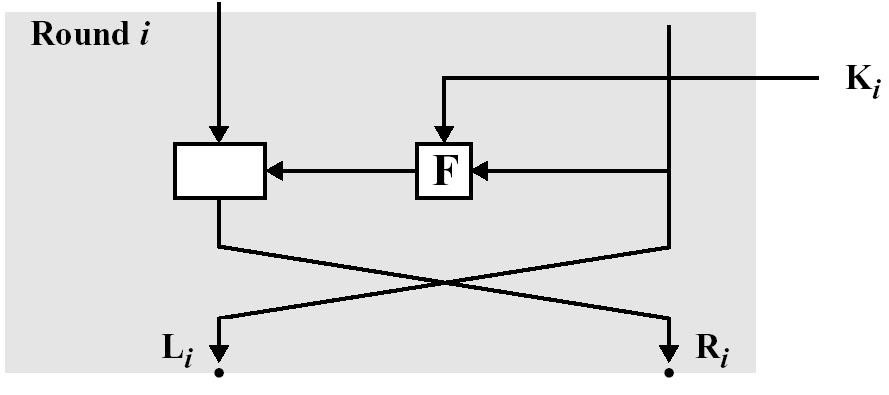# 密码学基础总结-04

## DES

### 菲斯特尔网络结构

• 明文分组分为$L_0$ 和$R_0$ ,通过$n$次循环处理后,再结合起来生成密文分组。
• 每$i$次循环都以上一循环产生的$L_{i-1}$和$R_{i-1}$ 和$K$产生的子密钥$K_i$作为输入。
• 所有循环的结构都相同,其方法是先对数据的右半部分和子密钥应用函数$F$,然后对函数输出结果和数据的左半部分取异或。
• 在置换之后,执行由数据两部分互换构成的交换。
• 解密过程与加密过程基本相同。规则如下:用密文作为算法的输入,但以相反顺序使用子密钥$K_i$。
• 加密和解密可以使用相同的方法。• 加密: $L_i = R_{i-1};R_i = L_{i-1} \oplus F(R_{i-1},K_{i})$
• 解密: $R_{i-1} = L_i;L_{i-1}=R_i \oplus F(R_{i-1},K_i) = R_i \oplus F(L_i,k_i)$